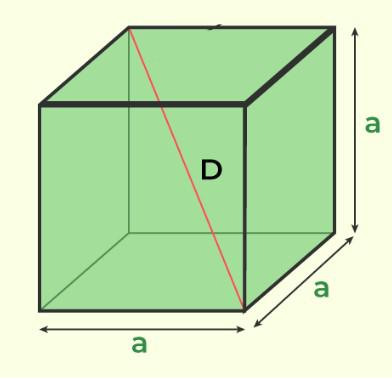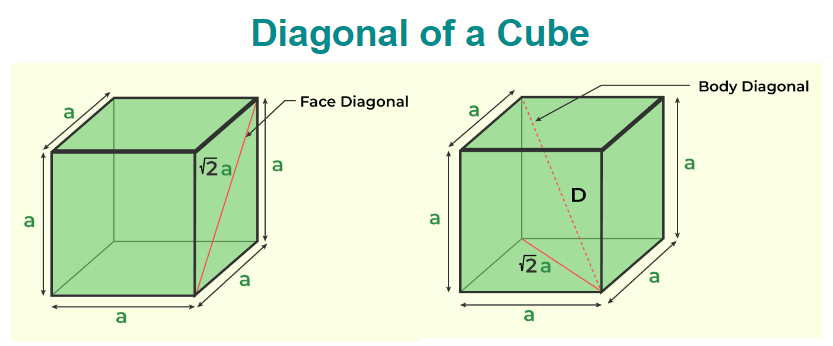Open In App

# Surface Area of a Cube Formula

Cube Formulas as the name suggest are all the formulas of a cube to calculate its volume, surface areas and diagonal. A cube is a three-dimensional figure that has equal length, breadth, and height. It is a special case of cuboid and is widely observed in our daily life. The most common example of the cube is the Rubick’s Cube puzzle. A cube has various formulas but the important formulas of the cube are,

• Volume of Cube Formula = a3
• Surface Area of Cube Formula = 6a2
• Diagonal of Cube Formula = �

where “a” is the side of the cube and is measured in the unit of length.

## What are Cube Formulas?

A cube is a 3-D object also called a regular hexahedron as it has six faces and the area of all the faces is equal. All the parameters of a cube, length, breadth, and height are equal. There are various formulas associated with a cube and the formulas of the cube are dependent on the side of the cube. The basic shape of the cube is added in the image below:There are three basic cube formulas that include,

• Surface Area of Cube formula
• Volume of Cube Formula
• Diagonal of Cube Formula

Let’s learn about these formulas in detail.

## Surface Area of Cube Formula

Surface Area of Cube is the area occupied by the faces of the cube. It depends on the side of the cube and is directly proportional to the square of the side of the cube. A cube has two types of areas that are,

• Lateral Surface Area of Cube
• Total Surface Area of Cube

### Lateral Surface Area of Cube Formula

Lateral Surface Area of a cube is the sum of the areas of the side faces of the cube. We know that a cube has four side faces of equal area and if the side of the cube is “a” then,

Area of One Face = a2

Lateral Surface Area(LSA) of Cube = 4 × Area of One Face

LSA of Cube = 4a2

### Total Surface Area of Cube Formula

Total Surface Area of a cube is the sum of the areas of all the faces of the cube. We know that a cube has a total of six faces four side faces and two top and bottom faces of equal area and if the side of the cube is “a” then,

Area of One Face = a2

Total Surface Area(TSA) of Cube = 6 × Area of One Face

TSA of a Cube = 6a2

Area or Surface Area of the cube is measured in (unit)2, i.e. in m2, cm2, etc.

## Volume of Cube Formula

The total capacity of a cube is called the volume of the cube. It is also defined as the total volume of the material used to make the solid cube. The volume of the cube is calculated by two formulas and they are,

Volume of a Cube = a3

where,

• a is the Length of the Side of a Cube

Volume of a Cube = 𢆣(d3)/9

where,

• d is the Length of the Diagonal of a Cube

Volume of the cube is measured in (unit)3, i.e. in m3, cm3, etc. It is also measured in other units also that include litre(l), millilitre(ml), etc.

## Diagonal of Cube Formula

Diagonal of the cube is the longest straight line that is drawn inside a cube. It is a dime of two joints two opposite vertices of the cube that are not in the same plane. A cube can have two diagonals, i.e.

• Body Diagonal
• Face Diagonal

The image added below shows the diagonal of the cube.### Body Diagonal

The body diagonal also called the main diagonal of the cube is a straight line that joints the two opposite vertices of the cube that are not on the same plane. It depends on the side of the cube and is directly proportional to it. This diagonal of the cube passes through its centre. If the side of the cube is “a”. Then its diagonal is calculated using

Diagonal of a Cube = �

### Face Diagonal

The Face diagonal of the cube is a straight line that joints the two opposite vertices of the cube that are on the same plane. It passes through the face of the cube and hence the name Face Diagonal. It depends on the side of the cube and is directly proportional to it. This diagonal of the cube passes through the centre of the face of the cube. If the side of the cube is “a”. Then its face diagonal is calculated using

Face Diagonal of a Cube = �

The diagonal of the cube is calculated using Pythagoras’ theorem and is measured in m, cm, etc.

## Solved Examples on Cube Formula

Example 1: Find the volume of a cube whose side is 5 cm.

Solution:

Given,

Side of Cube(s) = 5 cm

Volume of Cube(V) = s3 = s × s × s

V = (5)3 = 125 cm3

Thus, the volume of the cube is 125 cm3

Example 2: Find the surface area of the cube(TSA) whose side is 10 cm.

Solution:

Given,

Side of Cube(s) = 10 cm

TSA of Cube = 6s2

TSA = 6(10)2 = 6(100) cm2

TSA = 600 cm2

Thus, the total surface area of the cube is 600 cm2

Example 3: Find the body diagonal of the cube whose side is 27 m.

Solution:

Given,

Side of Cube(s) = 27 cm

Diagonal of Cube(d) = s𢆣

d = (27)𢆣

Thus, the diagonal of the cube is 27𢆣 m.

## FAQs on Cube Formula

### 1. What are Cube Formulas?

Various Cube Formula in Maths are,

• Volume of Cube Formula = a3
• Surface Area of Cube Formula = 6a2
• Diagonal of Cube Formula = �

where “a” is the side of the cube.

### 2. How to Calculate the Diagonal of a Cube?

To calculate the diagonal of the cube use the formula added below,

• Diagonal of Cube Formula = �

where “a” is side of the cube.

### 3. What is the Formula to Calculate the TSA of a Cube?

The formula used to caculate the TSA of a Cube,

• TSA of Cube Formula = 6a2

where “a” is side of the cube.

### 4. What is the Formula to Calculate the Volume(V) of a Cube?

The formula used to calculate the Volume of a Cube,

• Volume of Cube Formula = a3

where “a” is side of the cube.